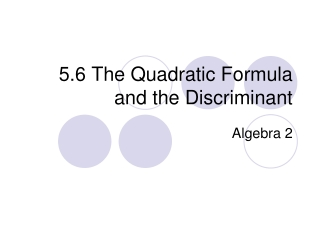DownloadDownload Presentation5.6 The Quadratic Formula and the Discriminant

# 5.6 The Quadratic Formula and the Discriminant

Télécharger la présentation## 5.6 The Quadratic Formula and the Discriminant

- - - - - - - - - - - - - - - - - - - - - - - - - - - E N D - - - - - - - - - - - - - - - - - - - - - - - - - - -
##### Presentation Transcript

1. Learning Target • Solve equations using the quadratic formula. • Use the discriminant to determine the nature of the roots of a quadratic equation.

2. Derive the quadratic formula from ax2 + bx + c = 0 a≠ 0 General form of a quadratic equation. Divide all by a Simplify Subtract c/a on both sides. Multiply by ½ and square the result.

3. Derive the quadratic formula from ax2 + bx + c = 0 a≠ 0 Add the result to both sides. Simplify Multiply by common denominator Simplify

4. Derive the quadratic formula from ax2 + bx + c = 0 a≠ 0 Square root both sides Simplify Common denominator/subtract from both sides Simplify

5. Quadratic Formula • The solutions of a quadratic equation of the form ax2 + bx + c with a ≠ 0 are given by this formula: MEMORIZE!!!!

6. Ex. 1: Solve t2 – 3t – 28 = 0 a = 1 b = -3 c = -28 There are 2 distinct roots—Real and rational.

7. CHECK: t2 – 3t – 28 = 0 72 – 3(7) – 28 = 0 49 – 21 – 28 = 0 49 – 49 = 0  CHECK: t2 – 3t – 28 = 0 (-4)2 – 3(-4) – 28 = 0 16 + 12 – 28 = 0 28 – 28 = 0  Ex. 1: Solve t2 – 3t – 28 = 0

8. Ex. 1: Solve t2 – 3t – 28 = 0 -- GRAPH

9. Ex. 2: Solve x2 – 8x + 16 = 0 a = 1 b = -8 c = 16 There is 1 distinct root—Real and rational.

10. Ex. 2: Solve x2 – 8x + 16 = 0 CHECK: x2 – 8x + 16 = 0 (4)2 – 8(4) + 16 = 0 16 – 32 + 16 = 0 32 – 32 = 0  There is 1 distinct root—Real and rational.

11. Ex. 2: Solve Solve x2 – 8x + 16 = 0 -- GRAPH

12. Ex. 3: Solve 3p2 – 5p + 9 = 0 a = 3 b = -5 c = 9 There is 2 imaginary roots.

13. Ex. 3: Solve 3p2 – 5p + 9 = 0 NOTICE THAT THE PARABOLA DOES NOT TOUCH THE X-AXIS.

14. Note: • These three examples demonstrate a pattern that is useful in determining the nature of the root of a quadratic equation. In the quadratic formula, the expression under the radical sign, b2 – 4ac is called the discriminant. The discriminant tells the nature of the roots of a quadratic equation.

15. DISCRIMINANT • The discriminant will tell you about the nature of the roots of a quadratic equation.

16. Ex. 4: Find the value of the discriminant of each equation and then describe the nature of its roots. 2x2 + x – 3 = 0 a = 2 b = 1 c = -3 b2 – 4ac = (1)2 – 4(2)(-3) = 1 + 24 = 25 The value of the discriminant is positive and a perfect square, so 2x2 + x – 3 = 0 has two real roots and they are rational.

17. Ex. 5: Find the value of the discriminant of each equation and then describe the nature of its roots. x2 + 8 = 0 a = 1 b = 0 c = 8 b2 – 4ac = (0)2 – 4(1)(8) = 0 – 32 = – 32 The value of the discriminant is negative, so x2 + 8 = 0 has two imaginary roots.

18. Pair-share • pp. 295 #16-45 even